# how to calculate WEEKNUMBER of a particular date in a yearHi Anaplanners,

i am stuck with one the calculation ,which requires me to calculate WEEK NUMBER of a date in the year.In excel we can use WEEKNUM Function to get the number of the week .

eg,WEEKNUM(2020-03-02) = 10

How can i achieve this

Tagged:

•Given you model calendar restraints I would recommend using the following approach;

Create a 'Time Properties - Year' module containing only time dimension set to Year. Add a single line item called, 'Start Date.'

Type the following formula - START()

This will populate the line item with the date of the first day of each calendar year.

Create a 'Time Properties - Day' module containing only time dimension but this time set to Day.

1. Date, format date, formula START()

1. WeekDay, format number, formula WEEKDAY(Date, 1) The '1' indicates the start of the week, Mon =1, Tue=2 etc

2. NewWeek, format number, formula IF WeekDay = 1 OR NewYear THEN 1 ELSE 0

3. NewYear, format boolean, formula Date = YEARVALUE('Time Properties - Year'.Start Date)

4. WeekNumber, format number, formula CUMULATE(New Week, NewYear)

Set all summary options to none.

The resulting table can be used to LOOKUP the week number for any given date within the model calendar depending on which day of the week each week starts. Adjust the WEEKDAY function to change this.

I have attached some screenshots to help you see what I have suggested.

Good luck,

Chris

•Create another line item formatted as TimePeriod - Weeks

Formula = PERIOD(Date) - Here Date is the Name of the First line Item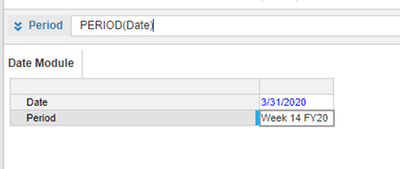•Hi @akprasa1,

You can achieve this using PERIOD() Function

Step 1: Line item - Date(Date Format), Week Number (Time Period - Week Format), and a number formatted line item.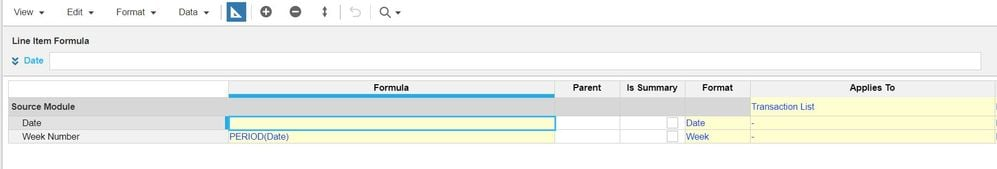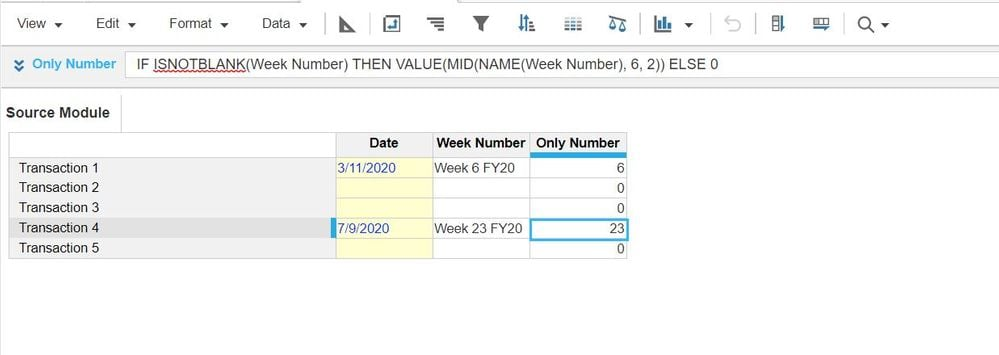Thanks,

Sorna Raja Prabhu

•Please do not clutter the formula.

VALUE(MID(NAME

Also do not hardcode the parameters like 6 here. There are tons of ways to make it dynamic esp using FIND function.

If you really need the Number only in Weeks then try and break the formula into multiple pieces/Line items. You will surely see a performance gain overall. Let's not neglect these small things, it takes a toll on model performance when the model scales up. Stick to Basics, take one function at a time in system modules.

Happy Modelling

•Hi @akprasa1 ,

Firstly, you need to check Week format as Numbered in Time Setting. if it is not then set as Numbered.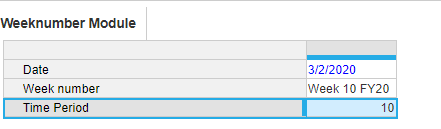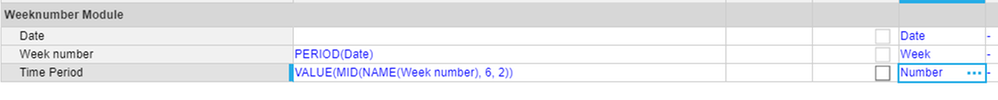Thanks,

•Thank you Misbah, for the suggestion.
•Hi Mishab,

Model Calendar is set to Calendar/Months/Years.

i will not be able to format the line items as time period - Week

•Model Calendar is set to Calendar/Months/Years.

i will not be able to format the line items as time period - Week

•Hi Sorna,

Model Calendar is set to Calendar/Months/Years.

i will not be able to format the line items as time period - Week

•Hi @akprasa1 ,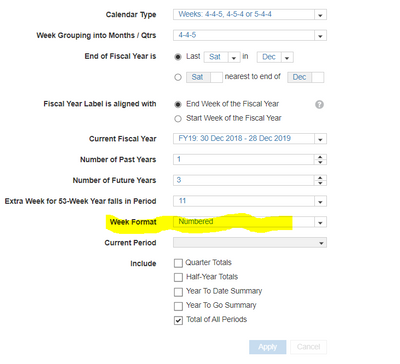Thanks,

•Hi All,

Model Calendar is set to Calendar/Months/Years in the model i am working with.

i will not be able to format the line items as time period - Week

•Hi @akprasa1 ,

Goto Blue Print view and scroll down to the right and click on Format Column then you will able to see Time Period.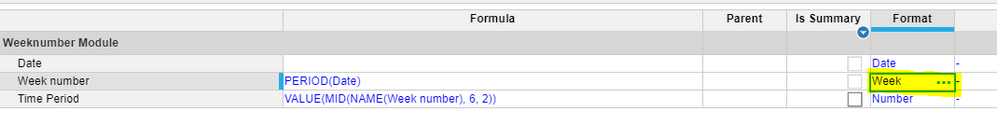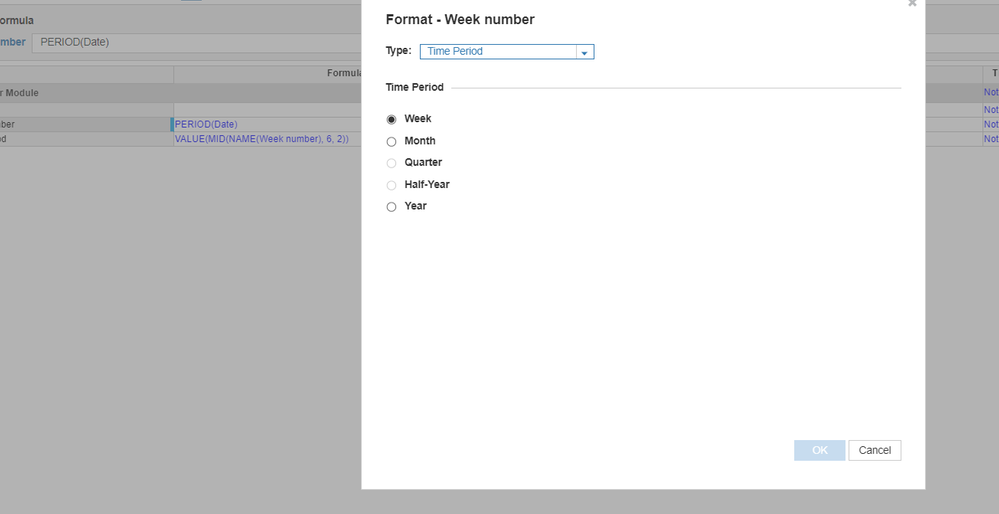•Looks like you did not get what i meant by my model calendar is set to Calendar/Months/Year.

I will not be able to select Week as a option.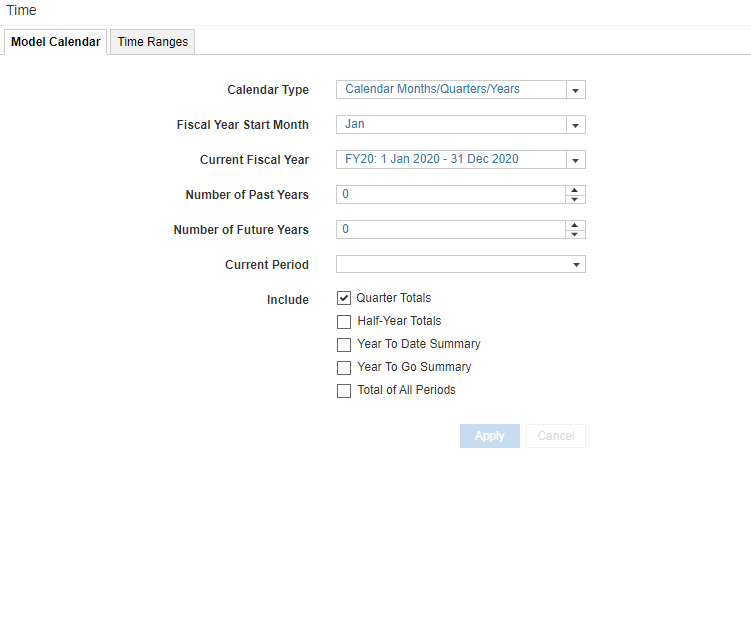Calendar is set to Months/Year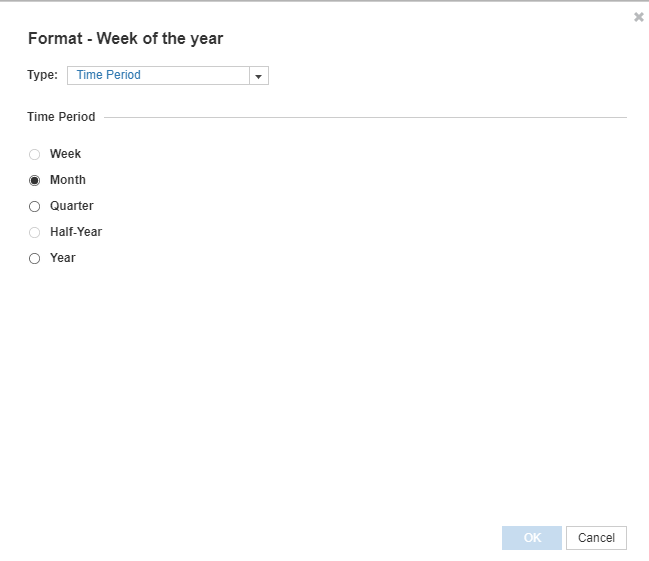Week selection is disabled

•In that case you will not be able to select Weeks and Anaplan too will not be able to show you Weekly Output of a date.

You will have to create a mapping module which can define the relationship between the dates and the weeks and use that mapping module for your reporting purposes.

•Nice one! But two things here

1. Your 53rd week ends with just 2 days.

2. When the model set up is at months, wouldn't it make much more sense to map the modules manually (one time set up) instead of letting Anaplan do the work for fake timescale . (There has to be a fake time scale somewhere if anything needs to be reported at week level)

Would love to hear your thoughts

•Hi @akprasa1,

I have another approach,

Step 1 : Create a System Module with 1 line item to manually enter the Calendar year's Starting date.

Eg; 29th December 2019 as First Staring day for calendar year 2020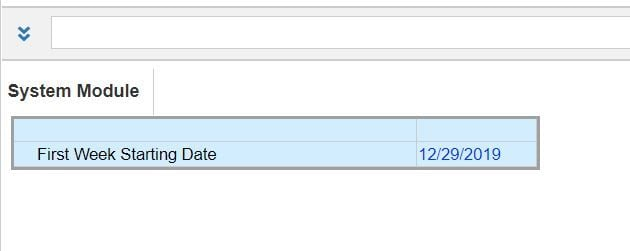Step 2:

in Your Main Module create 4 Line items.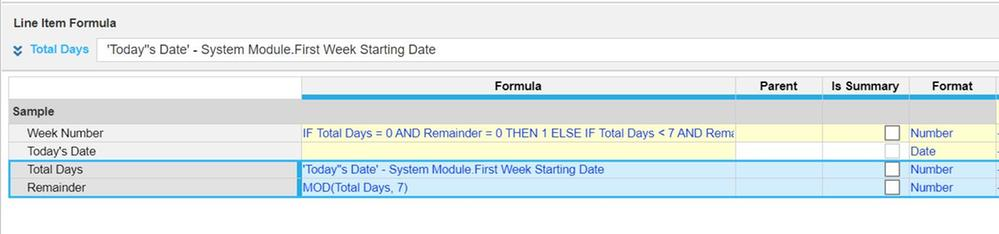Formulas are :

Today's Date : Manually enter the date, which you have to calculate the week for.

Total Days : 'Today''s Date' - System Module.First Week Starting Date (To Calculate number of days between Today's Date and Year Starting Date)

Remainder : MOD(Total Days, 7)

Week Number : IF Total Days = 0 AND Remainder = 0 THEN 1 ELSE IF Total Days < 7 AND Remainder < 7 THEN 1 ELSE IF Total Days >= 7 AND Remainder <> 6 THEN ROUND(Total Days / 7, 0, DOWN) + 1 ELSE ROUND(Total Days / 7)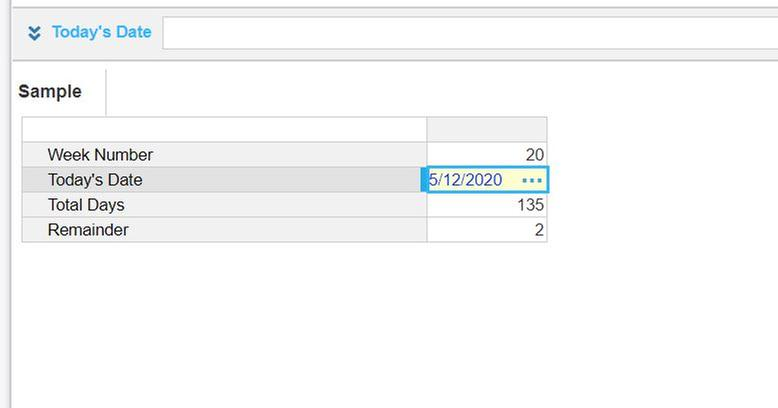easy logic -> let me know if u didn't understand.

Thanks,

Sorna Raja Prabhu

•My first thought was to create a manual mapping as you suggest but this would require ongoing maintenance which I try and avoid.

This approach will create two part weeks ( week 1 and week 53 ).
If the user wishes to create mapping with complete weeks then we need to fix the weekday number to equal that of the first day of the year.

We could achieve this by adding an extra line item in the Time Properties - Year module where we state what WeekDay number the first day of each year is.

If we amend the WeekDay and NewWeek formula above to reference this number rather than using a hard coded reference then the WeekNumber count will start from the first day of the year and increase by one every 7 days.

As you cant embed YEARVALUE into another function create a new line item in the Time Properties - Day module and use the following formula to reference the firs day of each new week.

YEARVALUE('Time Properties - Year'.DayNumber of new week)

•I have seen couple of solutions posted on this thread. I gave this a try, let me know if this is useful.

Things to consider:

Because the timescale in your model is at a monthly level, you can't specify when the week starts - on a Sunday/ Monday etc. In this case, I have assumed that the week starts on a Sunday. This can be changed based on your need.

The Structure:

You need 2 modules to identify the week number - one is a setup/ settings module, the other one is the core calc module.

Module - Year Start Calc:

Purpose of the module is to identify the start of the year based on the timescale. Current model timescale is from 1 Jan 2020 to 31 Dec 2020 at a monthly level. Now, 1 Jan 2020 is a Wednesday. Idea is to find the start of the week using the number of days in a week. Below is the structure and calc of this module: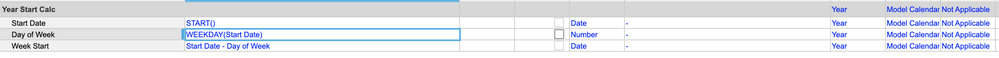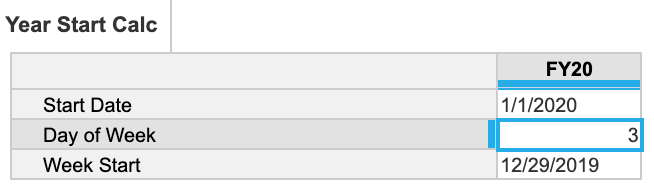You can club the 3 formulas in one if you wish to. I broke it down to show the flow of calc.

Module - Week Number Calculation:

Purpose of this module is to calculate the week number based on a date input. The first line item is for the input and rest 3 line items calculate the week number as desired.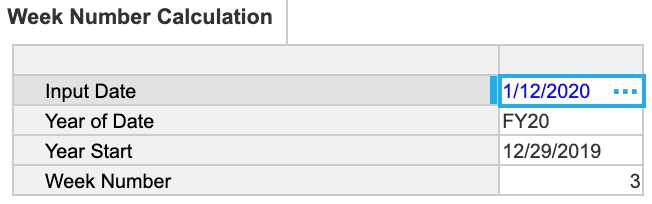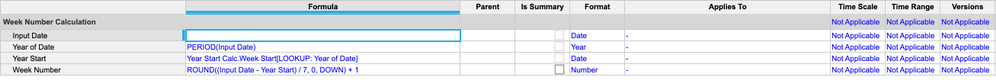I have tested with the dates in year 2020 and found it to be matching - let me know if you see any discrepancies.

This should scale if you increase the timescale to more years as it uses a lookup of year rather than a static input. Let me know if this helps.

Thanks

Anik

•The last formula is giving errors!